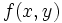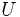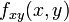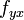# Clairaut's theorem on equality of mixed partials

(diff) ← Older revision | Latest revision (diff) | Newer revision → (diff)
Suppose$f$ is a real-valued function of two variables$x,y$ and$f(x,y)$ is defined on an open subset$U$ of$\R^2$. Suppose further that both the second-order mixed partial derivatives$f_{xy}(x,y)$ and$f_{yx}$ exist and are continuous on$U$. Then, we have:$\! f_{xy} = f_{yx}$
on all of$U$.